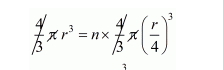# A spherical ball of iron has been melted and made into smaller balls.Question:

A spherical ball of iron has been melted and made into smaller balls. If the radius of each smaller ball is one-fourth of the radius of the original one, how many such balls can be made?

Solution:

Let radius of spherical ball = r

Then radius of smaller spherical ball $=\frac{r}{4}$

Let n be the no. of balls made by big spherical ball.

Clearly,

Volume of big spherical balls = n × volume of one smaller ball$r^{3}=n \times \frac{r^{3}}{64}$

$n=64$

Hence, the no. of balls = 64